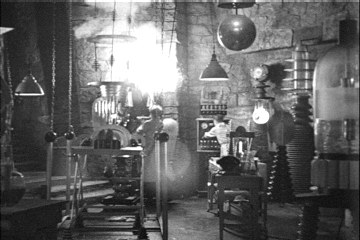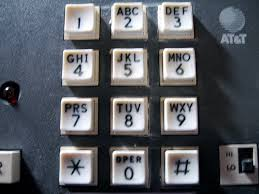# Programmation appliquée en ScalaCopyright © Cay S. Horstmann 2015# Writing a Higher-Order Function

• Let's write a function that checks whether all elements of a `Seq[Int]` fulfill a condition.
• `val forall = (s: Seq[Int], p: Int => Boolean) => ...`
• `p` is a function parameter
• `forall(1.to(10), _ > 0)` should return `true`
• `forall(1.to(10), _ % 2 == 0)` should return `false`
• We don't yet know how to do this efficiently, but we'll soldier on anyway:
`... => s.map(p) ...`
is a sequence of `true` or `false`
• We want all of them to be true:
`....reduceLeft(_ && _)`
• All together:
```val forall = (s: Seq[Int], p: Int => Boolean) =>
s.map(p).reduceLeft(_ && _)```

# What Does This Function Do?

```val mystery = (s: Seq[Int], p: Int => Boolean) =>
s.map(p).filter(_ == false).length == 0```
1. It returns `true` if all elements of `s` fulfill the predicate `p`
2. It returns `true` if at least one element of `s` fulfill the predicate `p`
3. It returns `true` if no elements of `s` fulfill the predicate `p`
4. Something else (none of the above, syntax error, etc.)

# Background: Comparison Functions

• To sort by a general criterion, specify how to compare elements
• In Scala, use a function `comp(s, t)` that returns `true` if `s` should come before `t`
• Different from C/Java (where a comparison function returns a value < 0, 0, > 0)
• ```val words = "Marianne had a little lamb".split(" ")
words.sortWith(_ < _) // Marianne, a, had, lamb, little
words.sortWith((s, t) => s.length < t.length) // a, had, lamb, little, Marianne
```
• What if we want to sort by the number of vowels?
```val isVowel = (c: Char) => "aeiouAEUOU".contains(c)
val vowels = (s: String) => s.filter(isVowel).length```
• No problem:
`words.sortWith((s, t) => vowels(s) < vowels(t))`
• That looks so similar to sorting by length. Can one avoid the repetition?

# Returning a Function

• Define a function that generates a comparator from a measurement (length, number of vowels, ...)
`val compareBy = (measure: String => Int) => ...`
• Need to return a function `(s: String, t: String) => ...`
• ...that checks that `measure(s) < measure(t)`
• All together:
```val compareBy = (measure: String => Int) =>
(s: String, t: String) => measure(s) < measure(t)
```
• ```words.sortWith(compareBy(vowels))
// had, a, lamb, little, Marianne```
• `words.sortWith(compareBy(_.length))`
• `compareBy` consumes a function and produces a function

# What Does `mystery` Do?

```val mystery = (
comp1: (String, String) => Boolean,
comp2: (String, String) => Boolean) =>
(s: String, t: String) =>
comp1(s, t) || !comp1(t, s) && comp2(s, t)
```

Sample usage:

`words.sortWith(mystery((s, t) => s.length < t.length, _ < _))`
1. `mystery` yields a comparison function that uses `comp2` to break ties if `comp1` doesn't distinguish between `s` and `t`
2. It yields a comparison function that considers `s` less than `t` if both `comp1` and `comp2` do
3. It yields a comparison function that considers `s` less than `t` if either `comp1` or `comp2` do
4. Something else (none of the above, syntax error, etc.)

# Lab• You work with a buddy
• One of you (the coder) writes the code, the other (the scribe) types up answers
• When you get stuck, ask your buddy first!
• Switch coder/scribe roles each lab
• The coder submits the worksheet. Include the scribe's name in the worksheet!
• The scribe submits answers. Include the coder's name in the report!

# Part 1: Defining Higher-Order Functions

1. Look up the Scaladoc for the `partition` method of `Seq`. You should implement an equivalent function that works for `Seq[Int]`.
2. ```val partition = (values: Seq[Int], p: Int => Boolean) => ...
```
3. What result do you get for `partition(1.to(10), _ % 3 == 1)`?
4. Write a function `rev` that reverses a comparator , so that, for example,
`words.sortWith(rev(compareBy(vowels))) `
yields the words with the most vowels first: `Marianne, little, ...`.
`val rev = (comp: (String, String) => Boolean) => (s: String, t: String) => ...`
Hint: This is completely trivial, but it takes some practice to think about it. You can't write `t < s` for `...` since that only works if `comp` happens to be `_ < _`. You need to express it in terms of `comp`.
5. Recall that
`words.sortWith(compareBy(vowels))`
yields
`had, a, lamb, little, Marianne`
What you expect to get when calling
`words.sortWith(rev(compareBy(vowels)))`
6. What did you actually get for `words.sortWith(rev(compareBy(vowels)))`? In which order did the strings with one vowel appear? Why didn't their positions get reversed?

# Part 2: Moving On with the Phone MnemonicsWe continue the “phone mnemonics” from Unit 3.

1. Have a closer look at the implementation of `wordsFor`. Inside it, you should have code that solves a more general problem where you have two vectors of strings and get a sequence of all concatenations from both of them. Write a function
`val cats = (s: Seq[String], t: Seq[String]) => ...`
that does this in general.
Test case:
`cats(Vector("Hello", "World"), Vector("Mary", "had", "fun"))`
2. Now write a function `wordsForDigits` that takes a string of digits between '2' and '9' and produces all strings that they can encode.
`val wordsForDigits = (digits: String) => ...`
Hint: First map to a vector of strings for each digit. Then use `reduceLeft`.
Test case: `wordsForDigits("72252")`
3. Read in all words from `/usr/share/dict/words`, as we did before. For efficiency, let's put them into a set though. And remove those that start with an uppercase letter (which include a bunch of junk) as well as those of length 1. And let's make them all uppercase. And let's add in "SCALA" which is weirdly missing.
`val words = io.Source.fromFile("/usr/share/dict/words").getLines.filter(w => Character.isLowerCase(w(0)) && w.length > 1).map(_.toUpperCase).toSet + "SCALA"`
Then improve the `wordsForDigits` function from the preceding step by filtering against the set.
4. Now when you run `wordsForDigits("72252")`, what do you get? What do you get for `wordsForDigits("72253")`?

# Part 3: Multiple Words

1. We've made a lot of progress, but we aren't quite there. The problem is a little harder. Given an arbitrary number like 7225247386, one is supposed to find the words "SCALA", "IS", "FUN" (and whatever other words there might be hidden).

Let's assume we have a particular way of breaking up the numbers, say into `Vector("72252", "47", "386")`. Using `wordsForDigits`, we get all the words for `"72252"`, `"47"`, and `"386"`. Now we want to concatenate them into a sentence.

We've almost solved that problem before, when `wordsForDigits` reduced with `cats` to glue together words. Give it a try:

```val wordsForDigitsSequence = (seq: Vector[String]) =>
seq.map(e => wordsForDigits(e)).reduceLeft(cats)```

What do you get for `wordsForDigitsSequence(Vector("72252", "47", "386"))?`

2. It would be easier to read the result if the words were separated by spaces. Make a copy of `cats`, call it `catsSpaces`, and make it separate the concatenated words by spaces. Then call `catsSpaces` instead of `cats` in `wordsForDigitsSequence`. What do you now get for `wordsForDigitsSequence(Vector("72252", "47", "386"))?`

3. We are doing good. If we know how to break up a number (such as 7225247386) into all corresponding sequences (such as 72252 47 386), then we can find the corresponding words for each sequence.

# Part 4: Breaking Up Is Hard to Do1. Let's find all ways that digits can be broken up into (non-empty) sequences. For example, the digits 1234 have 8 such decompositions: 1234, 12 34, 1 234, 1 2 34, 123 4, 12 3 4, 1 23 4, 1 2 3 4.

That looks pretty random, but it's not so bad. Suppose you knew how to break up 123 (into 123, 12 3, 1 23, 1 2 3). Now you augment that with 4. There are two things you can do:

• Add 4 all by itself: 123 4, 12 3 4, 1 23 4, 1 2 3 4
• Add 4 to the last element: 1234, 12 34, 1 234, 1 2 34

Let's do the first part.

`val augment1 = (a: Vector[Vector[String]], t: Char) => ...`

For each sequence `s` in `a`, you concatenate (with `++`) the sequence `Vector(t.toString)`.

Test case:

```augment1(Vector(Vector("123"),
Vector("12", "3"),
Vector("1", "23"),
Vector("1", "2", "3")),
'4')```
2. On to `augment2`. There are two inputs: a `Vector[Vector[String]]` such as `Vector(Vector("123"), Vector("12", "3"), Vector("1", "23"), Vector("1", "2", "3"))`, and a character such as `'4'` to glue to the last element of each sequence.

`val augment2 = (a: Vector[Vector[String]], t: Char) => ...`

For each sequence `s` in `a`, you concatenate (with `++`) all but the last element, followed by a `Vector(s.last + t)`

Test case:

```augment2(Vector(Vector("123"),
Vector("12", "3"),
Vector("1", "23"),
Vector("1", "2", "3")),
'4')```

3. Now define

`val augment = (a: Vector[Vector[String]], t: Char) => ...`

to concatenate `augment1(a, t)` and `augment2(a, t)` (with `++`).

Test case:

```augment(Vector(Vector("123"),
Vector("12", "3"),
Vector("1", "23"),
Vector("1", "2", "3")),
'4')```

4. What is the result of

`augment(augment(augment(Vector(Vector("1")), '2'), '3'), '4')`
5. This looks so promising. But why can't you use `augment` with `reduceLeft`?

Breaking up is hard to do. You'll have to wait until the next lecture to complete this puzzle.

# Homework

Do this as individual work, not with your partner

When all done, email the signed zip files to Fatemeh.Borran@heig-vd.ch